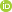«THE BULLETIN OF IRKUTSK STATE UNIVERSITY». SERIES «MATHEMATICS»
«IZVESTIYA IRKUTSKOGO GOSUDARSTVENNOGO UNIVERSITETA». SERIYA «MATEMATIKA»
ISSN 1997-7670 (Print)
ISSN 2541-8785 (Online)

## Multidimensional Exact Solutions of a System of Nonlinear Boussinesq Type Equations

Author(s)
A. A. Kosov,E. I. Semenov, V. V. Tirskikh
Abstract

We study the system of two nonlinear partial differential equations of the fourth order. The right parts of the system of equations contain multidimensional analogs of Boussinesq equation, expressed in terms of two-fold Laplace operators and squares of gradients of the required functions, as well as linear functions of the relationship. This kind of equations, similar to Navier-Stokes equations, encountered in problems of hydrodynamics. The paper proposes to search for a solution in the form of anzatz containing quadratic dependence on spatial variables and arbitrary functions on time. The use of the proposed anzatz allows to decompose the process of finding the solution components depending on the spatial variables and time. To find the dependence on spatial variables it is necessary to solve the algebraic system of matrix, vector and scalar equations. The General solution of this system of equations in parametric form is found. To find the time-dependent components of the solution of the initial system, a system of nonlinear ordinary differential equations arises. This system is reduced to one fourthorder equation for which particular solutions are found. A number of examples of the constructed exact solutions of the initial system of Boussinesq equations, including those expressed in terms of Jacobi functions in time and anisotropic in spatial variables, are given.

Alexander Kosov, Cand. Sci. (Phys.–Math.), Leading researcher; Matrosov Institute for System Dynamics and Control Theory of Siberian Branch of Russian Academy of Sciences (ISDCT SB RAS); Post Box 292, 134, Lermontov Str., Irkutsk, 664033, Russian Federation; tel.: (3952)427100, e-mail: kosov_idstu@mail.ru

Edward Semenov, Cand. Sci. (Phys.–Math.), Senior researcher; Matrosov Institute for System Dynamics and Control Theory of Siberian Branch of Russian Academy of Sciences (ISDCT SB RAS); Post Box 292, 134, Lermontov Str., Irkutsk, 664033, Russian Federation; tel.: (3952)453099, e-mail: edwseiz@gmail.com

Vladimir Tirskikh, Cand. Sci. (Phys.–Math.), Assoc. Prof., Irkutsk State Transport University, 15, Сhernyshevsky str., Irkutsk, 664074, Russian Federation; tel.: (3952)638311 e-mail: tirskikh_vv@irgups.ru

For citation

Kosov A.A., Semenov E.I., Tirskikh V.V. Multidimensional Exact Solutions of a System of Nonlinear Boussinesq Type Equations. The Bulletin of Irkutsk State University. Series Mathematics, 2019, vol. 30, pp. 114-124. (in Russian) https://doi.org/10.26516/1997-7670.2019.30.114

Keywords
nonlinear system, nonlinear Boussinesq equations, reduction, exact solutions
UDC
517.957
MSC
35L75
DOI
https://doi.org/10.26516/1997-7670.2019.30.114
References
1. Dodd R.K., Eilbeck J.C., Gibbon J.D., Morris H.C. Solitons and nonlinear waves equations. London, Academic Press, 1982, 630 p.
2. Kamke E. Differentialgleichungen: Losungsmethoden und Losungen I. Gewohnliche Differentialgleichungen. B.G. Teubner, Leipzig, 1977. https://dx.doi.org/10.1007/978-3-663-05925-7
3. Kosov A.A., Semenov E.I. Multidimensional exact solutions to the reaction-diffusion system with power-law nonlinear terms. Siberian Math. J., 2017, vol. 58, no. 4, pp. 619–632. https://doi.org/10.1134/S0037446617040085
4. Kosov A.A., Semenov E.I. On Exact Multidimensional Solutions of a Nonlinear System of Reaction–Diffusion Equations. Differential Equations, 2018, vol.54, no. 1, pp. 106-120. https://doi.org/10.1134/S0012266118010093
5. Kosov A.A., Semenov E.I., Tirskikh V.V. On Exact Multidimensional Solutions of a Nonlinear System of First Order Partial Differential Equation. The Bulletin of Irkutsk State University. Series Mathematics, 2019, vol. 28, pp. 53-68. https://doi.org/10.26516/1997-7670.2019.28.53
6. Pavlov M.V. Uravnenie Bussineska i preobrazovanie Miury [Boussinesq equation and Miura transform] Fundamental’naja i prikladnaja matematika [Fundamental and Applied Mathematics], 2004, vol. 10, no. 1, pp. 175-182. (in Russian)
7. Polyanin A.D., Zhurov A.I. Functional separable solutions of two classes of nonlinear mathematical physics equations. Doklady Mathematics, 2019, vol. 99, no. 3, pp. 321–324. https://doi.org/10.31857/S0869-56524863287-291
8. Polyanin A.D., Zaitsev V.F. Handbook of Nonlinear Partial Differential Equations. Chapman & Hall/CRC Press, Boca Raton, London, New York, 2012, 1912 p.
9. Polyanin A.D., Zaitsev V.F., Zhurov A.I. Metody resheniya nelinejnyx uravnenij matematicheskoj fiziki i mexaniki [Methods for solving nonlinear equations of mathematical physics and mechanics]. M.: Fizmatlit Publ., 2005, 256 p. (in Russian)
10. Skubachevskii A.L. Vlasov – Poisson equations for a two-component plasma in homogeneous magnetic field. Russian Math. Surveys, 2014, vol. 69, no. 2, pp. 291- 335. https://dx.doi.org/10.1070/RM2014v069n02ABEH004889
11. Galactionov V.A., Svirshchevskii S.R. Subspaces of nonlinear partial differential equations in mechanics and physics. Chapman & Hall/CRC, 2007, 493 p.
12. Markov Y., Rudykh G., Sidorov N., Sinitsyn A., Tolstonogov D. Steady state solutions of the Vlasov – Maxwell system and their stability. Acta Appl. Math., 1992, vol. 28, no. 3, pp. 253-293.
13. Sidorov N.A., Sinitsyn A.V. Stacionarnaya sistema vlasova-maksvella v ogranichennyx oblastyax [The stationary Vlasov – Maxwell system in bounded domains] "Nelinejnyj analiz i nelinejnye differencialnye uravneniya" [Nonlinear analysis and nonlinear differential equations]. Moscow, Fizmatlit Publ., 2003, pp. 50-88. (in Russian)